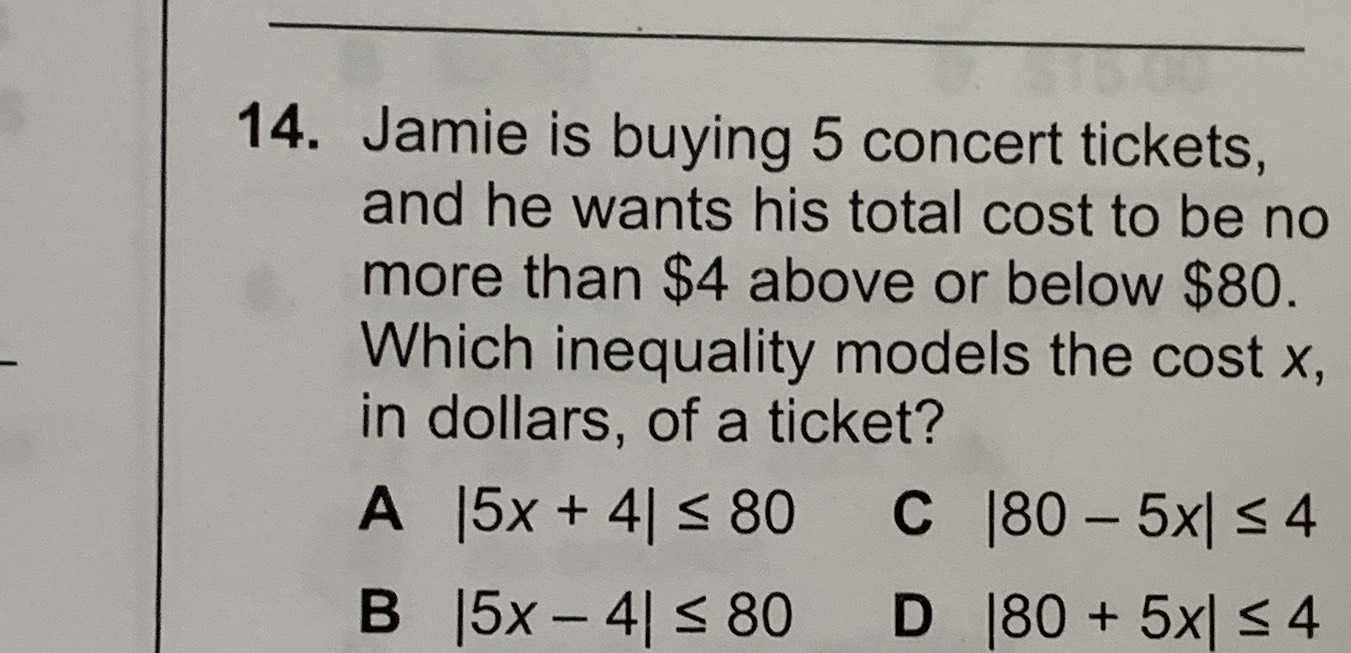### ¿Todavía tienes preguntas de matemáticas?

Pregunte a nuestros tutores expertos
Algebra
Pregunta14. Jamie is buying $$5$$ concert tickets, and he wants his total cost to be no more than $$\ 4$$ above or below $$\ 80 .$$ Which inequality models the cost $$x ,$$ in dollars, of a ticket?

$$| 5 x + 4 | \leq 80$$

$$| 5 x - 4 | \leq 80$$

$$| 80 - 5 x | \leq 4$$

$$| 80 + 5 x | \leq 4$$